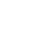0

# All possible pythagorean triples visualized

All possible pythagorean triples visualized

The theorem makes reference to a right-angled triangle such as that shown in Figure 1. The side opposite the right-angle is the longest side and is called the hypotenuse.What the theorem says is that the area of the square on the hypotenuse is equal to the sum of the areas of the squares on the two shorter sides. Figure 2 shows squares drawn on the hypotenuse and on the two shorter sides. The theorem tells us that area A + area B = area C.An excellent demonstration of this is available on the accompanying video. If we denote the lengths of the sides of the triangle as a, b and c, as shown, then area A = a 2 , area B = b 2 and area C = c 2 . So, using Pythagoras’ theorem area A + area B = area C a 2 + b 2 = c 2

Great #Animation #Video

## Like it? Share with your friends!

0hate
0
hateconfused
0
confusedfail
0
failfun
0
fungeeky
0
geekylove
0
lovelol
0
lolomg
0
omgwin
0
win